## Wednesday, April 29, 2020

### Unizor - Physics4Teens - Electromagnetism - Magnets

Notes to a video lecture on http://www.unizor.com

Magnetism - Magnets

We usually don't have a lot of questions about forces that act on a contact. There is an obvious cause and observable effect.

Forces that act on a distance cause a logical question "Why?" since
there is no obvious cause for observable actions, like attraction or
repelling of objects without immediate contact between them.

So far, we studied gravitational force, acting on a distance, and electrostatic force, also acting on a distance.

We have introduced a concept of a field to explain these actions on a distance and quantitatively study them.

In case of gravity we just stated that all objects have certain characteristic, mass, and any object has a gravitational field
around it that acts on any other object in this field, according to the
Law of Gravity. The force is always attracting and proportional to a mass. The gravitational force always exists around any object.

In case of electrostatics we explained the existence of a force on a distance and electrostatic field by an excess or deficiency of electrons, which we called an electric charge and assigned negative charge () to an excess of electrons and positive charge (+)
to their deficiency. In this case the force between two objects
positioned at a distance from each other can be either attracting (if
one object is positively charged and another - negatively, opposite
attracts) or repelling (for similarly charged objects, similar repels)
and is proportional to the number of electrons in excess or deficiency.

The electrostatic force exists only if there is an excess or deficiency
of electrons that usually requires certain effort to create by
separating certain electrons from their atoms. Electrostatic forces are
usually much stronger than gravitational.

There is another observable force on a distance. Certain objects, which are called permanent magnets,
have a force field around them that does not require any efforts to
create. This makes them similar to gravitation. But, while the force of
gravity is always attracting, magnets attract only some objects - those
that have certain magnetic properties.

The force field around magnets always exists and has an interesting property - polarity. Each magnet has two poles, which are conditionally called North and South.

Two magnets attract each other, if they are facing each other with
different poles, that is North pole of one magnet attracts South pole of
another (again, opposite attracts). Positioned to face each other by
the same pole (North to North or South to South), they repel each other.

Another interesting fact that differentiates magnets from electrically
charged objects is that magnets always have two poles - North and South.
There is no magnet with only North or only South pole. In the world of
electricity objects are usually either positively charged (deficiency of
electrons) or negatively charged (excess of electrons), not both at the
same time, but in the world of magnetism existence of two poles, North
and South, is a necessary property of every magnet.

Interestingly, our planet Earth is a giant magnet with North magnetic
pole positioned close to the geographical North pole (but not exactly at
it) and South magnetic pole positioned close to South geographical
pole. North and South magnetic poles of Earth are, actually, moving
because of movements of the planet core.

Compass is a device with a small magnet in a form a free rotating arrow,
also having North (usually, blue) and South (usually, red) poles. Since
opposite poles attract, the red part of a compass arrow (South pole of
an arrow magnet) points to the North magnetic pole of Earth.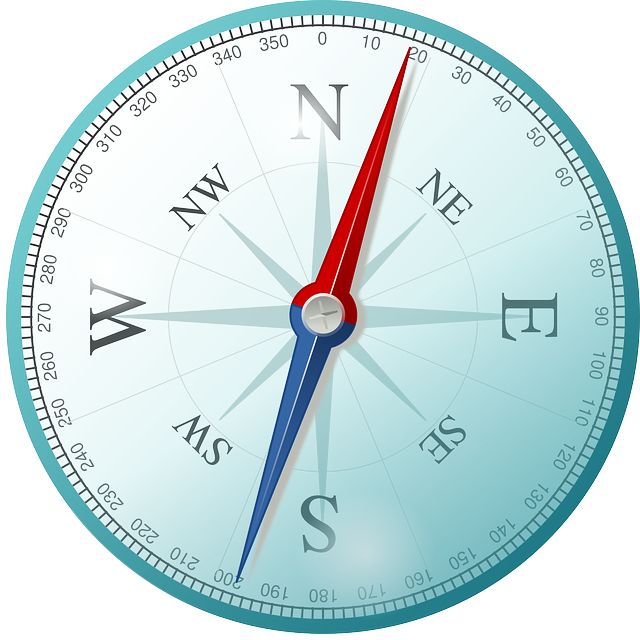In places far from Earth poles we can rely on a compass to determine the
general direction to the North. Close to the geographical poles a
compass is not a reliable tool, because the North and South magnetic
poles of Earth do not coincide with its geographical poles.

We do know that electric charge is caused by excess or deficiency of
electrons. This is a relatively superficial explanation. We never
discussed what caused two electrons to repel each other or proton and
electron to attract each other. These are much deeper issues that
require significantly more study.

With magnetism we also need some, however incomplete, explanation for this phenomenon.

Here is what might serve as a superficial explanation.

Electrons inside the atoms have their own magnetic properties, derived from their movement and so-called spin,
studied in Quantum Theory. Pair of electrons usually has electrons with
opposite spins and their magnetic poles neutralize each other. But in
case of odd number of electrons this balance is distorted. Atom with
such a magnetic unbalance becomes a source of magnetic field.

Another necessary property of the magnet is the relatively
unidirectional position of these atoms (all North poles are pointing to
the same direction), thus making the effect of magnetism much stronger.

## Friday, April 24, 2020

### Unizor - Physics4Teens - Electromagnetism - Direct Current - Electric He...

Notes to a video lecture on http://www.unizor.com

Direct Current - Electric Heat - Problems 1

Problem A

A lamp with resistance 500Ω (ohm) is powered by a source of electricity producing a voltage of 100V (volt).

What is the power consumption of this lamp?

Solution

Power is work per unit of time. It can be expressed as

P = U·I or

P = U² / R or

P = I²·R

Substituting the real numbers,

P = 100² / 500 = 20 (watt)

Problem B

Express the work performed by a source of electricity in Problem A during 8 hours in joules and in kilowatt-hours.

Solution

By definition,

1 watt = 1 joule/sec

Power consumed by a lamp is 20 watt (that is, 20 joules per second).

During 8 hours (that is, 8·3600 seconds) the work performed by a source of electricity will be equal to

W = 20·8·3600 = 576000 J (joules)

In kilowatt-hours (kWh) the work will be a product of power in kilowatts and time in hours, that is

W = 0.020·8 = 0.160 kWh

Problem C

There are usually two characteristics written on an incandescent
electric lamp: the difference in electric potential on the terminals of a
source of electricity the lamp is supposed to be connected to in volts (or voltage) and the power it consumes in watts (or wattage).

Let the voltage of a lamp be U=220V and the wattage - P=55W.

Determine the electric current I going through a lamp and its electrical resistance R.

Solution

Power is work per unit of time. It can be expressed as

P = U·I or

P = U² / R or

P = I²·R

From this, knowing U and P, we derive I and R.

I = P / U

R = U² / P

Substituting the real numbers,

I = 55 / 220 ≅ 0.25A (amperes)

R = 220²/55 = 880Ω (ohms)

Problem D

What electric current is supposed to go through an incandescent lamp with characteristics 110V, 55W, and what electric current will be going through it, if we connect it to a source of electricity with voltage 220V?

How much heat will be generated by this lamp in this case of abnormally high voltage?

Solution

P = U·I = I²·R = U²/R

R = U²/P

R = (110V)²/(55V) = 220Ω

Inorm = (55W)/(110V) = 0.5A

Ihigh = (220V)/(220Ω) = 1A

Phigh = (220V)²/(220Ω) = 220W

In this case of high voltage the lamp will generate 220W of heat, which is 4 times greater amount of heat than normal. It will burn very soon.

Problem E

Two incandescent lamps are connected in series to a source of electricity with voltage 220V.

The first lamp has characteristic 220V and 55W.

The second lamp has characteristic 110V and 55W.

What electric current will be going through these lamps along a circuit?

Will the amount of heat produced by each lamp be greater or less than the one it's designed to produce?

Solution

P = U·I = I²·R = U²/R

R = U²/P

R1 = 220²/55 = 880Ω

R2 = 110²/55 = 220Ω

Rtotal = R1 + R2 = 1100Ω

I = U/Rtotal = 220/1100 = 0.2A

P1 = 0.2²·880 = 35.2W

Consumed power of 35.2W is less than 55W, it will produce less light than it is manufactured for.

P2 = 0.2²·220 = 8.8W

Consumed power of 8.8W is significantly less than 55W, it will be hardly lit at all.

Problem F

Two incandescent lamps are connected in parallel to a source of electricity with voltage 220V.

The first lamp has characteristic 220V and 55W.

The second lamp has characteristic 110V and 55W.

What electric current will be going through a common part of a circuit?

Will the amount of heat produced by each lamb be greater or less than the one it's designed to produce?

Solution

P = U·I = I²·R = U²/R

R = U²/P

R1 = 220²/55 = 880Ω

R2 = 110²/55 = 220Ω

Rtotal = 1 / (1/R1 + 1/R2) = 176Ω

Icommon = U/Rtotal = 220/176 = 1.25A

P1 = 220²/880 = 55W

Consumed power of 55W is exactly the same as normal, it will produce exactly the same amount of heat and light as it is manufactured for

P2 = 220²/220 = 220W

Consumed power of 220W is 4 times greater than normal 55W, it will produced a lot of heat and light, but will burn soon.

## Sunday, April 19, 2020

### Unizor - Physics4Teens - Electromagnetism - Electric Heat

Notes to a video lecture on http://www.unizor.com

Direct Current - Electric Heat

Consider a circuit with a source of electricity that produces a difference in electric potential (voltage) U(volts) between its terminals. The only other component in this simple circuit is a resistor with resistance R(ohms).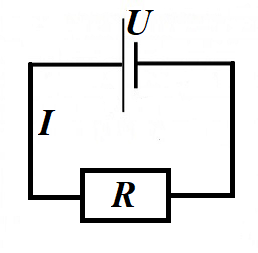According to the Ohm's Law, the electric current going through a resistor is

I = U/R(amperes)

Recall that a difference in electric potential (voltage) of 1V(one volt) between two terminals of a source of electricity means that it requires one joule of work (1J) to move one coulomb of positive electric charge (+1C) between these terminals.

Therefore,

1V·1C = 1J and 1V = 1J/1C

Further recall that the electric current of 1A(one ampere) is the flow of electricity, when 1C(one coulomb) of electricity is moving across the wire within 1sec(one second).
Therefore,

1A = 1C/1sec and 1A·1sec = 1C

In case of our circuit with the voltage between the terminals of a source of electricity U, electric current I and resistor R we have I coulombs of electric charge going through a circuit each second.

So, it t seconds the source of electricity moves I·t coulombs of electricity.

Moving one coulomb (1C) of electricity between terminals with one volt (1V) of a difference in electric potential requires one joule (1J) of work.

Therefore, moving this one coulomb (1C) of electricity between terminals with a difference of electric potential (voltage) U volts requires U joules of work.

Continuing this logic, moving I·t coulombs of electricity between the terminals with the voltage U volts requires U·I·t joules of work.

So, the work performed by a source of electricity with voltage U (volts) that moves I coulombs electricity every second (amperage) through a resistor with resistance R ohms during time t sec is (in joules)

W = U·I·t

From the Ohm's Law U=I·R immediately follows

W = I²·R·t and W = U²·t / R

In most cases we will assume that wires have zero resistance and only
resistors have the property of resistance. So, in our simple circuit the
only resistance to the moving electrons is in a resistor R.

Since the source of electricity performs work, this work is supposed to
do something. According to the Law of Energy Conservation, the work is
just a transformation of one form of energy into another.

In our case the only result of the work performed by a source of
electricity is moving electrons in a circuit that, meeting resistance of
the atoms inside a resistor, push them around, thus increasing their
chaotic movement inside a resistor. This chaotic movement is heat that can be measured, for example, by measuring a temperature of a resistor.

The bottom line is that a source of electricity that has voltage V, producing an electric current with amperage I going through a resistor with resistance R during time t performs work

W = U·I·t = I²·R·t = U²·t / R

which is converted to heat that increases the temperature of a resistor.

This process is used in, for example, in incandescent lamps. The rising
temperature of a tungsten spiral inside such a lamp results in heat and
light radiation that lights and heats up the space around a lamp.

## Saturday, April 11, 2020

### Unizor - Physics4Teens - Electromagnetism - DC Ohm's Law - Problems 3

Notes to a video lecture on http://www.unizor.com

Direct Current - Ohm's Law -
Problems 3

Problem A
Consider the following circuit with the voltage U and all resistances given.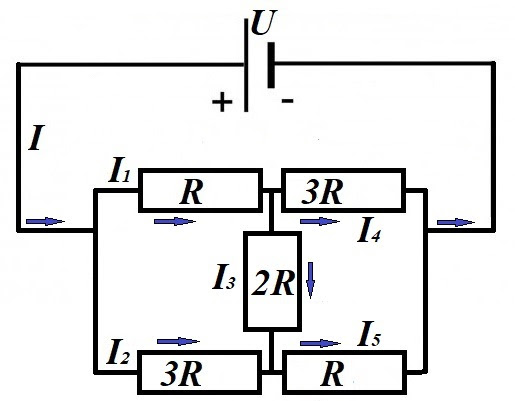Ignore for now the arrows on the picture, they will be explained in the solution.
The task is to determine all the electric currents I1I2I3I4I5 going through each resistor and the current I in the common segment of the circuit.

Solution
This problem differs from seemingly analogous one presented before. There is no symmetry here, no points with the same electric potential that can be connected or disconnected for convenience.
We will solve it using the most general brute force approach.

First of all, we will randomly choose the direction of the unknown electric currents in each segment of a circuit. Randomly, because we don't really know the direction, except in the common segment with current I. In this segment the direction of current is from a positive terminal of the battery to a negative one (as you recall, by definition, it's opposite to the flow of electrons). In other cases we cannot determine the direction, and we choose any particular one. After the calculations it might be positive (if we correctly choose the direction) or negative (if in reality the direction is opposite).

We have six unknown values of the electric current in this problem. These values are related to each other because they have to obey certain laws of Physics, which we will use to have sufficient number of equations to find algebraically these unknown values.

Next, let's use the principle of balance of the current at every connection. Amount of electricity going into this connection per unit of time must be equal to amount electricity going out of it.
I = I1 + I2
I1 = I3 + I4
I2 + I3 = I5
I4 + I5 = I

To add more equations (we need six), let's consider a voltage drop on any part of a circuit with the same chosen direction of the current.

Going along the top of the circuit through resistors with resistances R and 3R, the combined voltage drop should be equal to a voltage between terminals of a battery
I1·R + I4·(3R) = U

Going along the bottom of the circuit through resistors with resistances 3R and R, the combined voltage drop should also be equal to a voltage between terminals of a battery
I2·(3R) + I5·R = U

Going along the top of the circuit through resistor with resistances R, then down through resistor 2R and right through resistor R, the combined voltage drop should also be equal to a voltage between terminals of a battery
I2·R + I3·(2R) + I5·R = U

Going along the bottom of the circuit through resistor with resistances 3R, then up through resistor 2R (against the current, so we should subtract the voltage drop) and right through resistor 3R, the combined voltage drop should also be equal to a voltage between terminals of a battery
I2·(3R) − I3·(2R) + I4·(3R) = U

We have got eight linear equations, with only six unknowns, which means that our system contains dependent equations, but it's OK, it can be algebraically solved.

Solution by substitution
(long by straight forward):

I=x,
I1=y,
I2=z,
I3=u,
I4=v,
I5=w,
A=U/R

x=y+z
y=u+v
z+u=w
v+w=x
y+3v=A
3z+w=A
y+2u+w=A
3z-2u+3v=A
______________
w=z+u
x=y+z
y=u+v
v+(z+u)=x
y+3v=A
3z+(z+u)=A
y+2u+(z+u)=A
3z-2u+3v=A
______________
x=y+z
y=u+v
v+z+u=x
y+3v=A
4z+u=A
y+3u+z=A
3z-2u+3v=A
______________
v=y-u
x=y+z
v+z+u=x
y+3v=A
4z+u=A
y+3u+z=A
3z-2u+3v=A
______________
x=y+z
(y-u)+z+u=x
y+3(y-u)=A
4z+u=A
y+3u+z=A
3z-2u+3(y-u)=A
______________
u=A-4z
x=y+z
y+z=x
same as above
y+3(y-u)=A
y+3u+z=A
3z-2u+3(y-u)=A
______________
x=y+z
4y-3(A-4z)=A
y+3(A-4z)+z=A
3z-5(A-4z)+3y=A
______________
z=x-y
4y+12z=4A
y-11z=-2A
3y+23z=6A
______________
3x-2y=A
-11x+12y=-2A
23x-20y=6A
______________
2y=3x-A
-11x+12y=-2A
23x-20y=6A
______________
-11x+6(3x-A)=-2A
23x-10(3x-A)=6A
______________
7x=4A
-7x=-4A
same as above
______________
x=4A/7
y=(12A/7 - A)/2 = 5A/14
z=3A/14 u=A-12A/14 = A/7
v=5A/14 - A/7 = 3A/14
w=3A/14 + A/7 = 5A/14
______________
I = 4U/(7R)
I1 = 5U/(14R)
I2 = 3U/(14R)
I3 = U/(7R)
I4 = 3U/(14R)
I5 = 5U/(14R)

Notice the anti-symmetry in resistors (top left is the same as bottom right R, top right is the same as bottom left 3R).
It results in equality of the corresponding currents:
I1 = I5,
I2 = I4.
______________

Knowing the current
I=4U/(7R),
we can calculate the total resistance of the circuit using the Ohm's Law:
Rcircuit = U/I = (7/4)·R

## Sunday, April 5, 2020

### Unizor - Physics4Teens - Electromagnetism - DC Ohm's Law - Problems 2

Notes to a video lecture on http://www.unizor.com

Direct Current - Ohm's Law -
Problems 2

Problem A
Two resistors R1 and R2 are connected parallel to each other in a circuit.
Prove that the currents flowing through them are inversely proportional to their resistances, that is I1/I2=R2/R1.

Hint
It follows directly from the Ohm's Law.

Problem B
Given a square ABCD with diagonal AC.
Each side of this square and diagonal AC are resistors with resistance R each.
Points A and D are connected to a source of electricity with voltage U.
Determine resistance of an entire circuit RABCD and current IAC along the diagonal AC.

Solution
Redraw the circuit as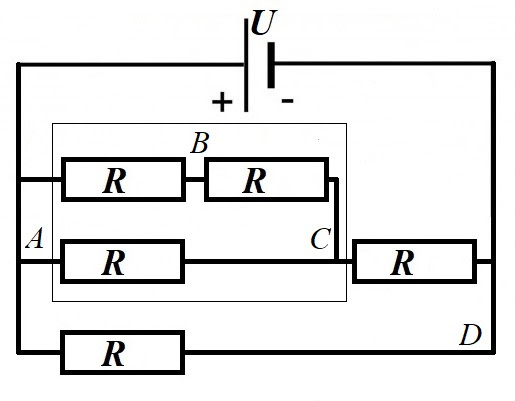and start with calculating the resistance of elements in a thin rectangle.
1/RABC = 1/R + 1/(2R) = 3/(2R)
RABC = 2R/3
Now the total resistance of a circuit is calculated as a parallel connection of the resitors with resistance (2R/3)+R=5R/3 at the top branch and another R at the bottom of a drawing
1/RABCD = [1/(5R/3)] + 1/R =
= 3/(5R) + 1/R = 8/(5R)

Total resistance of a circuit is
RABCD = 5R/8
Current from the source of electricity in the common part of a circuit is, therefore,
I = 8U/(5R)
To find the current along diagonal AC, we need to know the voltage between points A and C.
The voltage between points A and D is U.
The current in a common wire to the top branch with resistance 5R/3 is, therefore,
Itop = 3U/(5R)
The voltage in that branch drops from point A to point C by
UABC = Itop·RABC =
= 3U/(5R) · 2R/3 = 2U/5

Therefore, the current in the diagonal AC is
IAC =
RABCD = 5R/8
IAC = 2U/(5R)

Problem C
Given a cube ABCDEFGH made with identical resistors R on each edge, 12 resistors altogether.
It is connected to the source of electricity at two vertices on its main diagonal AG.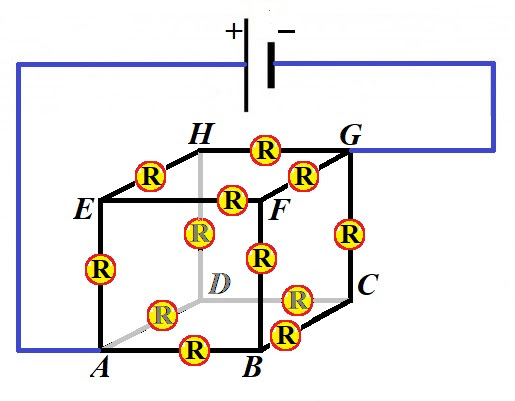What is the total resistance Rcube of this cube?

Solution
The cube is a symmetrical figure relatively to its main diagonal AG and, since all resistors are the same, one on each edge, we can use this consideration to state that the main current from the source of electricity to point A is divided into three identical currents along edges ABAD and AE.
Therefore, a voltage drop at points BD and E is exactly the same and there is no difference in electric potential between these three vertices.
If there is no difference in electric potential between points BD and E, there will be no movement of electrons between these three points if we merge these three points into one point X. The flow of electricity will not change by this merging. Analogously, the main current from the source of electricity to point G is divided into three identical currents along edges GCGF and GH.
Therefore, a voltage drop at points CF and H is exactly the same and there is no difference in electric potential between these three vertices.
If there is no difference in electric potential between points CF and H, there will be no movement of electrons between these three points if we merge these three points into one point Y. The flow of electricity will not change by this merging.
The following picture is the result of this transformation.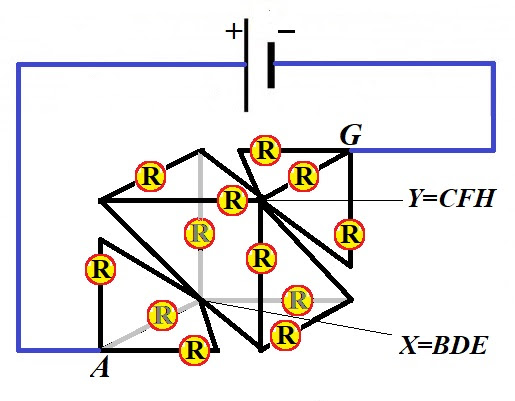Regardless of seemingly complex picture, it actually represents a simple circuit.
Between points A and X there are three identical parallel resistors. Their combined resistance is RAX.
RAX = R/3
Between points X and Y there are six identical parallel resistors. Their combined resistance is RXY.
RXY = R/6
Between points Y and G there are three identical parallel resistors. Their combined resistance is RYG.
RYG = R/3
These three groups are connected in a series with combined resistance
Rcube = R/3 + R/6 R/3 = 5R/6

## Saturday, April 4, 2020

### Unizor - Physics4Teens - Electromagnetism - DC Ohm's Law - Problems 1

Notes to a video lecture on http://www.unizor.com

Direct Current - Ohm's Law -
Problems 1

Problem A
Determine currents II1 and I2, if voltage at the source of electric power U and resistances R1R2 and R3 are given.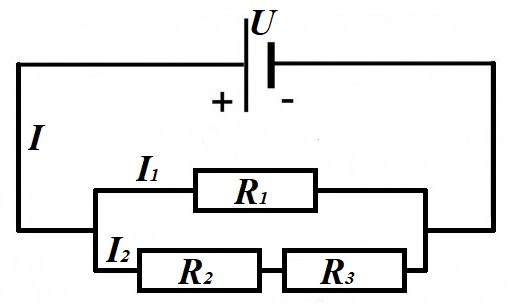Solution
R23 = R2 + R3
1/R = 1/R1 + 1/R23
I = U/R
I1 = U/R1
I2 = U/R23

Problem B
Consider a circuit that contains the source of electric field with difference of electric potential on its terminals (voltageU and a resistor with resistance R. The electric field forces the electrons to flow through the circuit, which means the field performs some work.
Express the work performed by an electric field during time t and its power in terms of voltage U, current I and resistance R.

Solution
Assume that q coulombs of electricity flows through a circuit during time t.
Using the definition of the difference of potentials (voltageU and the definition of the electric current I=q/t, the work performed by a field A equals to
A = U·q = U·I·t
and from the Ohm's Law I=U/R or U=I·R, the latter can be written as
A = I²·R·t = U²·t/R
From the definition of power as work performed in a unit of time W=A/t follows
W = I²·R = U²/R

Problem C
The Energy Conservation Law dictates that the work performed by an electric field to move electrons around a circuit defined by voltage Uamperage I and resistance R should result in something tangible.
This tangible result is the heat. The molecules inside the resistor are pushed around by electrons circulated in the circuit and, as a result, the temperature of the resistor increases.
The amount of heat produced in the resistor equals to amount of work performed by the electric field (Joule-Lenz Law).
Consider the circuit with a resistor being a regular incandescent lamp with a spiral made of tungsten with mass m=0.001kg.
The spiral resistance is R=200Ω.
The voltage produced by a source of electric field is 110V.
Tungsten specific heat capacity is c=134J/(kg·K).
We switch on the electricity in a circuit for the period of t=2sec.
What will be the rise in temperature of the tungsten spiral at the end?

Solution
Work performed by an electric field:
A = U²·t/R
(same as amount of heat inside the resistor)
The amount of heat is
Q = 110²·2/200 = 121 joules
Heat Q, mass m, change of temperature ΔT and specific heat c are connected by the following law:
Q = c·m·ΔT
Therefore,
ΔT = Q / (c·m)
Using the values given,
ΔT = 121/(134·0.001) = 903°K
This will be the rise of temperature after 2 sec of working electricity.

Problem D
Determine currents II1 and I2, if voltage at the source of electric power U and resistance R are given.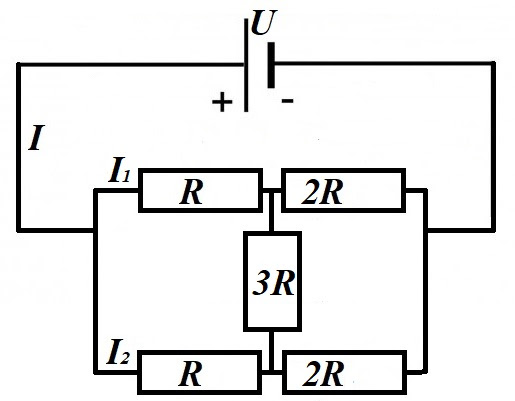Solution
The problem with this circuit is that it cannot be considered as consisting from parallel and series resistors. The resistor 3R, connecting top and bottom branches of a circuit, prevents it.
However, notice that the circuit is symmetrical, its top part (resistors R and 2R) is the same as the bottom part. From this symmetry we conclude that
I1 = I2 = I/2
and the voltage drops on resistors with resistance R on the top and on the bottom are the same
ΔU = (I/2)·R
Therefore, the difference in electric potentials at the ends of resistor 3R is zero and there is no electric current going through it, and it can be removed and excluded from all the calculations.
What's left after we exclude resistor 3R from consideration is parallel connection of two pairs of resistors connected in a series.
Total resistance of each branch (top or bottom) is a sum of two resistors R and 2R connected in a series
Rbranch = R + 2R = 3R
Total resistance of two branches together is calculated from a formula for parallel connection of two resistors with resistance 3R each
1/Rtotal = 1/(3R) + 1/(3R)
Rtotal = 3R/2
Now we can calculated the electric current I from the Ohm's Law
I = U/Rtotal = 2U/(3R)
I1 = I2 = I/2 = U/(3R)

### Unizor - Physics4Teens - Ohm's Law - Batteries, Generators

Notes to a video lecture on http://www.unizor.com

Direct Current -
Batteries, Generators

In talking about electricity we will often use examples with heat distribution.
Consider a house with a heating system.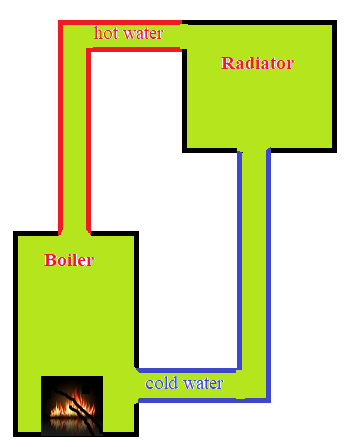A radiator, connected to a boiler by pipes, is installed in a room. The boiler heats up the water that goes from its outgoing pipe to an intake of a radiator, where it releases its heat, goes out of a radiator and comes back to a boiler intake pipe. There it is heated again and a circle continues.

The boiler produces energy (heat), pipes transport it to a radiator, where it's released to a room.

With an electric circuit that contains some source of electric energy (battery, generator etc.) and a resistor the process is very similar.
Inside a source of energy some electrons are separated from their nuclei and sent to one terminal, which becomes negatively charged, while those atoms that lost some of their electrons are sent to the other terminal, which becomes positively charged.
This process generates potential energy, since positive and negative charges are attracted to each other, and a battery separates them and does not let them to connect back within it.

If we have a circuit from one terminal to one end of a resistor and from another end of a resistor to another terminal of the source of electric energy, the flow of electrons resembles the flow of water through a pipe from the boiler to a radiator, heating the room and flowing back to the boiler. The water in the radiator, heating the room, is like electrons making their way through a resistor, hitting its atoms that resist the movement of electrons. The water pipes are like wiring that connects a resistor to the source of electric energy. The boiler is like a source of electric energy (a battery or a generator). Full analogy.

The potential energy of positive and negative charges in presence of a circuit is transformed into kinetic energy of electrons moving along a circuit, partially spending its energy on dragging through atoms of resistor and ending up on the positive terminal of the battery.
A simple mechanical equivalent is the process of a child climbing up a ladder to the top of a slide, thus generating a potential energy and, instead of going back down the stairs, slides down a slope, partially spending kinetic energy to friction.

Using the analogy of heater and radiator, let's analyze what happens if we have more then one source of electricity. In particular, what happens if we connect batteries in a series or parallel to each other.

Series Connection

Consider first the situation with two boilers connected in a series. That is, outgoing pipe with hot water from one boiler goes to another boiler, which adds more heat to the water before it's sent from it to a radiator, from which the water goes back to the intake of the first radiator.

Obviously, the water, being heated twice, will be more hot than heated only once. So, the amount of water going to a radiator will be the same as with one boiler, but its temperature will be greater.

Similar process occurs when two batteries are connected in a series as follows: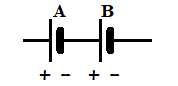Battery A is capable of separating certain number of electrons from their nuclei, so does battery B.
When connected in a series, battery B separates its own electrons plus is given the already separated electrons form battery A, thus increasing the number of electrons separated from their nuclei. That creates a larger difference in potential between positive terminal of A and negative terminal of B.

Therefore, batteries in a series increase the difference of potential (voltage) between their free ends.
For example, if a single AA battery provides 1.5V on its terminals, two such batteries connected in a series provide 3V.

This type of connection in a series is used when we connect 2 standard AA batteries to power TV remote control that requires 3V to work properly. Or we connect 6 such batteries in a series to get 9V difference in potentials to power a toy car.

So, all batteries connected in a series work at their usual capacity, last exactly as if they work separately, just the voltage is increased.

Conclusion: The voltage of an assembly of several sources of electric power connected in series is a sum of all individual voltages of its components.

Parallel Connection

Again, let's start with boiler analogy. Consider two identical boilers connected parallel to each other, that is outgoing pipes with hot water from both of them are joined and go to a radiator, where the hot water releases its heat, cools down, and the cold water on its way out is split into both intakes of two boilers.

Under these conditions the temperature of the hot water is the same as if only one boiler worked. At the same time, each boiler can work with half of its power because the total amount of hot water needed to heat the room is now split into two boilers.

Now consider two identical batteries connected parallel to each other.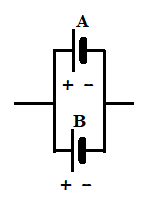The difference in electric potential (voltage) on the free ends of this assembly is the same as the voltage on the ends of each battery. So, the voltage in parallel connection remains the same.

At the same time the electrons needed by electric power consumer (a lamp, a refrigerator etc.) connected into a circuit with these batteries will consume half of needed electrons from one battery and another half from another.
So, the batteries in parallel connection share the burden and will work longer. Actually, about twice as long in this configuration than if only one battery supplied the power to a device that consumes the electricity.

For example, if a single AA battery provides 1.5V on its terminals and works for 1 hour supplying electricity to a small lamp, two such batteries connected in parallel provide the same 1.5V for this lamp, but will last about 2 hours.